# Compute spatial resolution metrics to compare MEG with EEG+MEG#

Compute peak localisation error and spatial deviation for the point-spread functions of dSPM and MNE. Plot their distributions and difference of distributions. This example mimics some results from 1, namely Figure 3 (peak localisation error for PSFs, L2-MNE vs dSPM) and Figure 4 (spatial deviation for PSFs, L2-MNE vs dSPM). It shows that combining MEG with EEG reduces the point-spread function and increases the spatial resolution of source imaging, especially for deeper sources.

```# Author: Olaf Hauk <olaf.hauk@mrc-cbu.cam.ac.uk>
#
```
```import mne
from mne.datasets import sample
from mne.minimum_norm.resolution_matrix import make_inverse_resolution_matrix
from mne.minimum_norm.spatial_resolution import resolution_metrics

print(__doc__)

data_path = sample.data_path()
subjects_dir = data_path / "subjects/"
meg_path = data_path / "MEG" / "sample"
fname_fwd_emeg = meg_path / "sample_audvis-meg-eeg-oct-6-fwd.fif"
fname_cov = meg_path / "sample_audvis-cov.fif"
fname_evo = meg_path / "sample_audvis-ave.fif"

# read forward solution with EEG and MEG
# forward operator with fixed source orientations
forward_emeg = mne.convert_forward_solution(
forward_emeg, surf_ori=True, force_fixed=True
)

# create a forward solution with MEG only
forward_meg = mne.pick_types_forward(forward_emeg, meg=True, eeg=False)

# noise covariance matrix

# evoked data for info

# make inverse operator from forward solution for MEG and EEGMEG
inv_emeg = mne.minimum_norm.make_inverse_operator(
info=evoked.info, forward=forward_emeg, noise_cov=noise_cov, loose=0.0, depth=None
)

inv_meg = mne.minimum_norm.make_inverse_operator(
info=evoked.info, forward=forward_meg, noise_cov=noise_cov, loose=0.0, depth=None
)

# regularisation parameter
snr = 3.0
lambda2 = 1.0 / snr**2
```
```Reading forward solution from /home/circleci/mne_data/MNE-sample-data/MEG/sample/sample_audvis-meg-eeg-oct-6-fwd.fif...
Computing patch statistics...
[done]
Computing patch statistics...
[done]
Desired named matrix (kind = 3523) not available
Read MEG forward solution (7498 sources, 306 channels, free orientations)
Desired named matrix (kind = 3523) not available
Read EEG forward solution (7498 sources, 60 channels, free orientations)
Forward solutions combined: MEG, EEG
Source spaces transformed to the forward solution coordinate frame
Average patch normals will be employed in the rotation to the local surface coordinates....
Converting to surface-based source orientations...
[done]
306 out of 366 channels remain after picking
366 x 366 full covariance (kind = 1) found.
Read a total of 4 projection items:
PCA-v1 (1 x 102) active
PCA-v2 (1 x 102) active
PCA-v3 (1 x 102) active
Average EEG reference (1 x 60) active
Read a total of 4 projection items:
PCA-v1 (1 x 102) active
PCA-v2 (1 x 102) active
PCA-v3 (1 x 102) active
Average EEG reference (1 x 60) active
Found the data of interest:
t =    -199.80 ...     499.49 ms (Left Auditory)
0 CTF compensation matrices available
nave = 55 - aspect type = 100
Projections have already been applied. Setting proj attribute to True.
No baseline correction applied
Computing inverse operator with 364 channels.
364 out of 366 channels remain after picking
Selected 364 channels
Whitening the forward solution.
Created an SSP operator (subspace dimension = 4)
Computing rank from covariance with rank=None
Using tolerance 3.3e-13 (2.2e-16 eps * 305 dim * 4.8  max singular value)
Estimated rank (mag + grad): 302
MEG: rank 302 computed from 305 data channels with 3 projectors
Using tolerance 4.7e-14 (2.2e-16 eps * 59 dim * 3.6  max singular value)
Estimated rank (eeg): 58
EEG: rank 58 computed from 59 data channels with 1 projector
Setting small MEG eigenvalues to zero (without PCA)
Setting small EEG eigenvalues to zero (without PCA)
Creating the source covariance matrix
Computing SVD of whitened and weighted lead field matrix.
largest singular value = 4.31738
scaling factor to adjust the trace = 1.68644e+20 (nchan = 364 nzero = 4)
Computing inverse operator with 305 channels.
305 out of 306 channels remain after picking
Selected 305 channels
Whitening the forward solution.
Created an SSP operator (subspace dimension = 3)
Computing rank from covariance with rank=None
Using tolerance 3.3e-13 (2.2e-16 eps * 305 dim * 4.8  max singular value)
Estimated rank (mag + grad): 302
MEG: rank 302 computed from 305 data channels with 3 projectors
Setting small MEG eigenvalues to zero (without PCA)
Creating the source covariance matrix
Computing SVD of whitened and weighted lead field matrix.
largest singular value = 4.63485
scaling factor to adjust the trace = 1.05542e+20 (nchan = 305 nzero = 3)
```

## EEGMEG#

Compute resolution matrices, localization error, and spatial deviations for MNE:

```rm_emeg = make_inverse_resolution_matrix(
forward_emeg, inv_emeg, method="MNE", lambda2=lambda2
)
ple_psf_emeg = resolution_metrics(
rm_emeg, inv_emeg["src"], function="psf", metric="peak_err"
)
sd_psf_emeg = resolution_metrics(
rm_emeg, inv_emeg["src"], function="psf", metric="sd_ext"
)
del rm_emeg
```
```    364 out of 366 channels remain after picking
Preparing the inverse operator for use...
Scaled noise and source covariance from nave = 1 to nave = 1
Created the regularized inverter
Created an SSP operator (subspace dimension = 4)
Created the whitener using a noise covariance matrix with rank 360 (4 small eigenvalues omitted)
Applying inverse operator to ""...
Picked 364 channels from the data
Computing inverse...
Eigenleads need to be weighted ...
Computing residual...
Explained  43.2% variance
[done]
Dimension of Inverse Matrix: (7498, 364)
Dimensions of resolution matrix: 7498 by 7498.
```

## MEG#

Do the same for MEG:

```rm_meg = make_inverse_resolution_matrix(
forward_meg, inv_meg, method="MNE", lambda2=lambda2
)
ple_psf_meg = resolution_metrics(
rm_meg, inv_meg["src"], function="psf", metric="peak_err"
)
sd_psf_meg = resolution_metrics(rm_meg, inv_meg["src"], function="psf", metric="sd_ext")
del rm_meg
```
```    305 out of 306 channels remain after picking
Preparing the inverse operator for use...
Scaled noise and source covariance from nave = 1 to nave = 1
Created the regularized inverter
Created an SSP operator (subspace dimension = 3)
Created the whitener using a noise covariance matrix with rank 302 (3 small eigenvalues omitted)
Applying inverse operator to ""...
Picked 305 channels from the data
Computing inverse...
Eigenleads need to be weighted ...
Computing residual...
Explained  46.7% variance
[done]
Dimension of Inverse Matrix: (7498, 305)
Dimensions of resolution matrix: 7498 by 7498.
```

## Visualization#

Look at peak localisation error (PLE) across the whole cortex for PSF:

```brain_ple_emeg = ple_psf_emeg.plot(
"sample",
"inflated",
"lh",
subjects_dir=subjects_dir,
figure=1,
clim=dict(kind="value", lims=(0, 2, 4)),
)

brain_ple_emeg.add_text(0.1, 0.9, "PLE PSF EMEG", "title", font_size=16)
```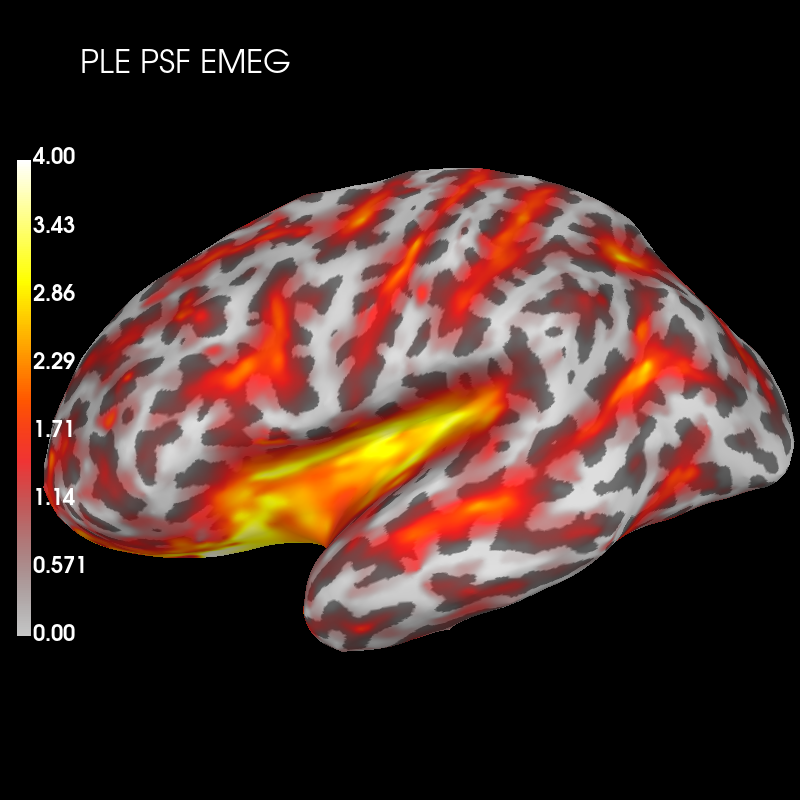For MEG only:

```brain_ple_meg = ple_psf_meg.plot(
"sample",
"inflated",
"lh",
subjects_dir=subjects_dir,
figure=2,
clim=dict(kind="value", lims=(0, 2, 4)),
)

brain_ple_meg.add_text(0.1, 0.9, "PLE PSF MEG", "title", font_size=16)
```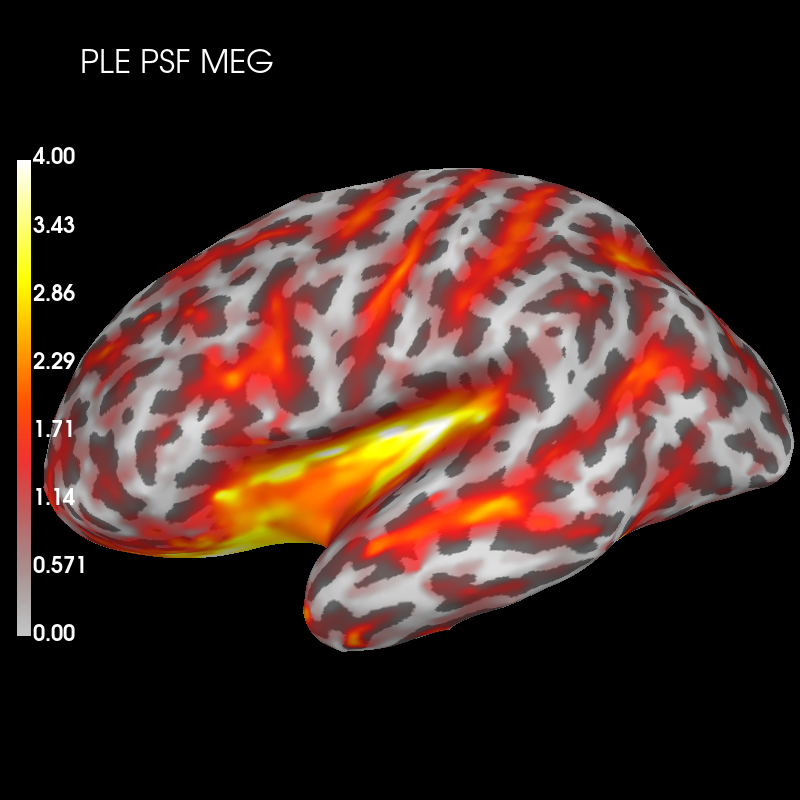Subtract the two distributions and plot this difference:

```diff_ple = ple_psf_emeg - ple_psf_meg

brain_ple_diff = diff_ple.plot(
"sample",
"inflated",
"lh",
subjects_dir=subjects_dir,
figure=3,
clim=dict(kind="value", pos_lims=(0.0, 0.5, 1.0)),
smoothing_steps=20,
)

brain_ple_diff.add_text(0.1, 0.9, "PLE EMEG-MEG", "title", font_size=16)
```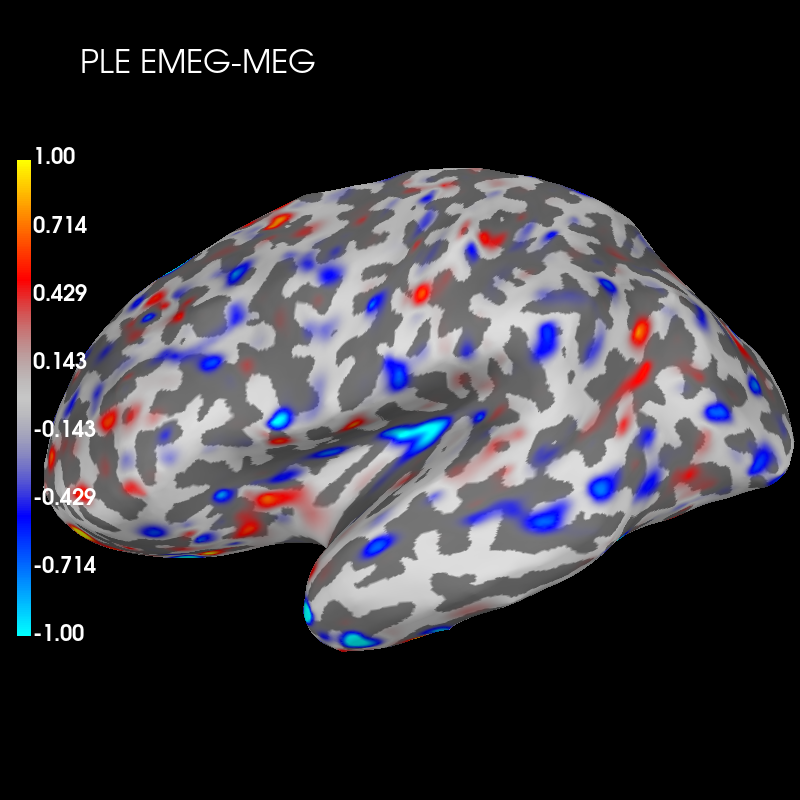These plots show that with respect to peak localization error, adding EEG to MEG does not bring much benefit. Next let’s visualise spatial deviation (SD) across the whole cortex for PSF:

```brain_sd_emeg = sd_psf_emeg.plot(
"sample",
"inflated",
"lh",
subjects_dir=subjects_dir,
figure=4,
clim=dict(kind="value", lims=(0, 2, 4)),
)

brain_sd_emeg.add_text(0.1, 0.9, "SD PSF EMEG", "title", font_size=16)
```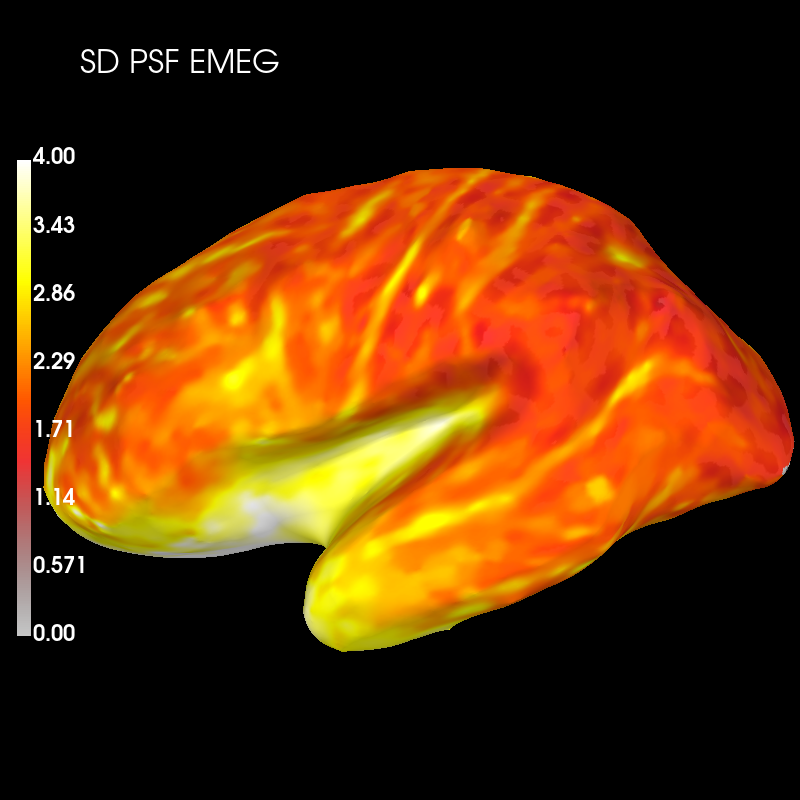For MEG only:

```brain_sd_meg = sd_psf_meg.plot(
"sample",
"inflated",
"lh",
subjects_dir=subjects_dir,
figure=5,
clim=dict(kind="value", lims=(0, 2, 4)),
)

brain_sd_meg.add_text(0.1, 0.9, "SD PSF MEG", "title", font_size=16)
```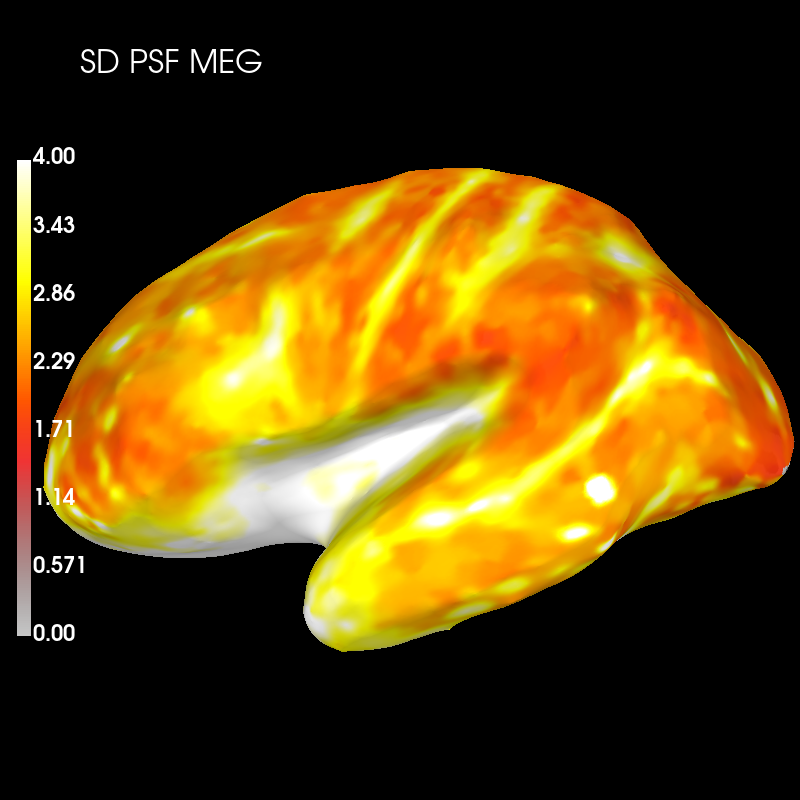Subtract the two distributions and plot this difference:

```diff_sd = sd_psf_emeg - sd_psf_meg

brain_sd_diff = diff_sd.plot(
"sample",
"inflated",
"lh",
subjects_dir=subjects_dir,
figure=6,
clim=dict(kind="value", pos_lims=(0.0, 0.5, 1.0)),
smoothing_steps=20,
)

brain_sd_diff.add_text(0.1, 0.9, "SD EMEG-MEG", "title", font_size=16)
```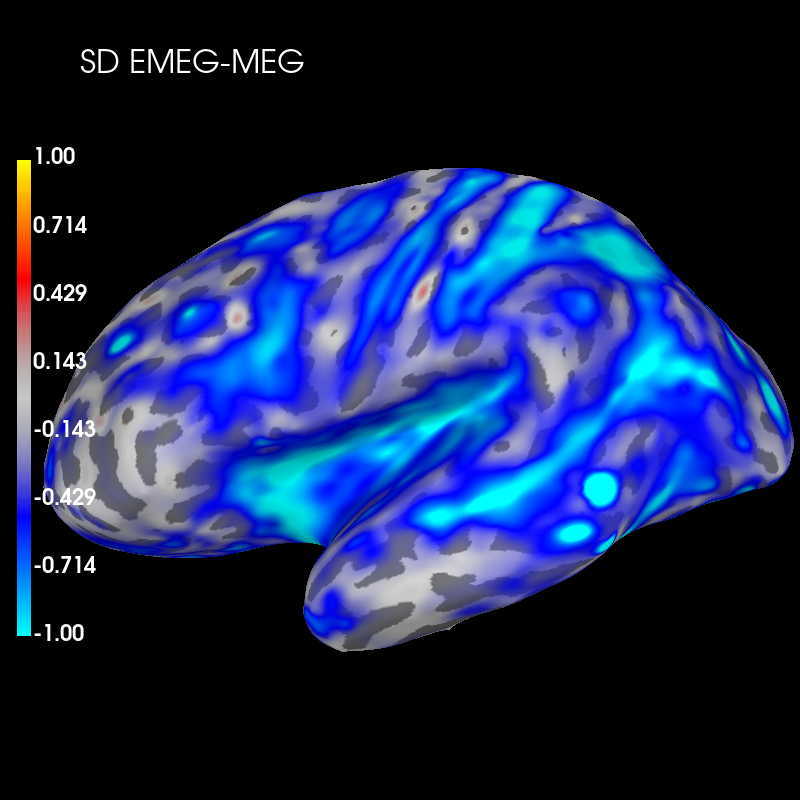Adding EEG to MEG decreases the spatial extent of point-spread functions (lower spatial deviation, blue colors), thus increasing resolution, especially for deeper source locations.